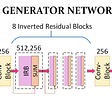# Review — Non-local Neural Networks (Video Classification)

## Space, Time, & Spacetime Long-Range Interactions Captured by Non-local Neural Networks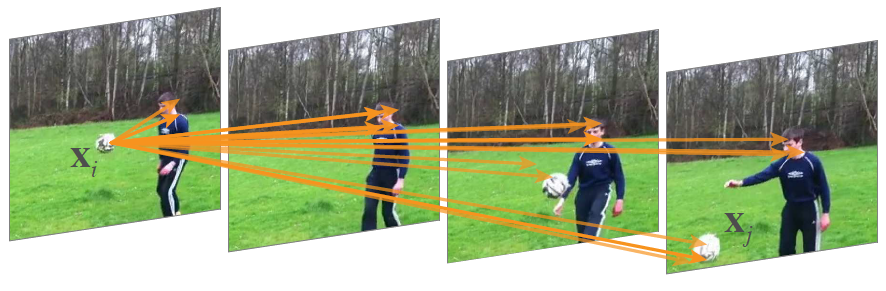A spacetime non-local operation in our network trained for video classification in Kinetics. In this example computed by the proposed model, note how it relates the ball in the first frame to the ball in the last 2 frames.

In this story, Non-local Neural Networks, (NL), by Carnegie Mellon University, and Facebook AI Research, is reviewed. In this paper:

• The non-local operation computes the response at a position as a weighted sum of the features at all positions.
• It is used for Video Classification, Object Detection, Instance Segmentation, and Human Pose Estimation (Keypoint Detection) tasks.

This is a paper in 2018 CVPR with over 3100 citations. (Sik-Ho Tsang @ Medium)

# Outline

1. Motivation of Non-Local Blocks
2. Generic Definition of Non-Local Blocks
3. Instantiations of Non-Local Blocks
4. Non-Local Blocks
5. Ablation Study on Video Classification
6. SOTA Comparison on Video Classification
7. Experimental Results on COCO

# 1. Motivation of Non-Local Blocks

In videos, long-range interactions occur between distant pixels in space as well as time.

A single non-local block, which is the basic unit, can directly capture these spacetime dependencies in a feedforward fashion.

• With a few non-local blocks, the architectures called non-local neural networks are more accurate for video classification than 2D and 3D convolutional networks
• In addition, non-local neural networks are more computationally economical than the 3D convolutional counterparts.

# 2. Generic Definition of Non-Local Blocks

## 2.1. Generic Definition

• A generic non-local operation in deep neural networks:
• Here, i is the index of an output position (in space, time, or spacetime) whose response is to be computed depending on the task.
• j is the index that enumerates all possible positions.
• x is the input signal (image, sequence, video; often their features).
• y is the output signal of the same size as x.
• A pairwise function f computes a scalar (representing relationship such as affinity) between i and all j.
• The unary function g computes a representation of the input signal at the position j.
• The response is normalized by a factor C(x).

The non-local behavior in the above equation is due to the fact that all positions (∀j) are considered in the operation.

• The above equation supports inputs of variable sizes, and maintains the corresponding size in the output.

A non-local operation is a flexible building block and can be easily used together with convolutional/recurrent layers, build a richer hierarchy that combines both non-local and local information.

## 2.2. Differences From Convolutional, Recurrent, Fully Connected Operations

• As a comparison, a convolutional operation sums up the weighted input in a local neighborhood, i−1≤ ji+1 when kernel size is 3.
• A recurrent operation at time i is often based only on the current and the latest time steps (e.g., j = i or i−1).
• The non-local operation is also different from a fully-connected (fc) layer. The above equation computes responses based on relationships between different locations, whereas fc uses learned weights.

# 3. Instantiationsof Non-Local Blocks

• Recap the above equation:
• There can be several versions of f and g, which will be discussed below.

## 3.1. Unary Function g(xj)

• For simplicity, g is only considered in the form of a linear embedding:
• where Wg is a weight matrix to be learned.
• Then, it is 1×1 convolution in space or 1×1×1 convolution in spacetime.

## 3.2.1. Gaussian

• Following non-local mean and bilateral filter approaches, a natural choice of f is the Gaussian function. In this paper, f is considered as:
• Here xiTxj is dot-product similarity.
• The normalization factor is set as:

## 3.2.2. Embedded Gaussian

• A simple extension of the Gaussian function is to compute similarity in an embedding space.
• where θ and ϕ are two embeddings:
• The normalization factor is the same:
• Self-attention module proposed in a very famous paper “Attention is all you need” , is a special case of non-local operations in the embedded Gaussian version.
• It can be seen that for a given i, the softmax computation along the dimension j, becomes:
• That means, we got:

In this work, it gives the insight by relating this recent self-attention model to the classic computer vision method of non-local means.

It also extends the sequential self-attention network in  to a generic space/spacetime non-local network for image/video recognition in computer vision.

• (If interested, please read the paper: Attention is all you need.)
• But there can be another 2 alternative versions instead of using softmax, as describe below, i.e. dot product and concatenation.

## 3.2.3. Dot Product

• f can be defined as a dot-product similarity using embedded version:
• where the normalization factor is the number of positions in x:
• It simplifies gradient computation. A normalization like this is necessary because the input can have variable size.
• The main difference between the dot product and embedded Gaussian versions is the presence of softmax.

## 3.2.4. Concatenation

• f also can be a concatenation form:
• where [·, ·] denotes concatenation and wf is a weight vector that projects the concatenated vector to a scalar.
• The normalization factor is:

# 4. Non-Local Blocks

• By wrapping the equation of:
• into a non-local block, we can define the non-local block as:
• where “+xi” is the residual connection.
• The pairwise computation as described in the previous section (Section 3) can be simply done by matrix multiplication.
• This pairwise computation as done by matrix multiplication is comparable to a typical convolutional layer in standard networks.
• The concatenation version is straightforward.
• The pairwise computation of a non-local block is lightweight.

## 4.1. An Illustrative Example

• For example, typical values in the above figure are T=4, H=W=14 or 7.
• As the example in the figure above, the shape of their tensors, e.g., T×H×W×1024 for 1024 channels.
• The blue boxes denote 1×1×1 convolutions.
• The embedded Gaussian version, is with a bottleneck of 512 channels.
• The bottleneck design of Wg, and is to reduce the computation of a block by about a half.
• The vanilla Gaussian version can be done by removing θ and ϕ.
• The dot-product version can be done by replacing softmax with scaling by 1/N.
• The weight matrix Wz computes a position-wise embedding on yi, matching the number of channels to that of x.

## 4.2. Non-Local Blocks for Subsampling

• A subsampling trick cane be done by using subsampled version of x, i.e. ^x:
• It can be done by pooling.

# 5. Ablation Study on Video Classification

• Kinetics dataset contains 246k training videos and 20k validation videos. It is a classification task involving 400 human action categories.
• ImageNet pretrained ResNet-50 C2D (2D convolution) is used as baseline.
• The input video clip has 32 frames each with 224×224 pixels.
• This model processes the input frame-by-frame, the only operation involving the temporal domain are the pooling layers.

## 5.1. Instantiations

• Different types of a single non-local block added to the C2D baseline (right before the last residual block of res4).
• Interestingly, the embedded Gaussian, dot-product, and concatenation versions perform similarly, up to some random variations (72.7 to 72.9).
• The non-local operations with Gaussian kernels become similar to the self-attention module.
• The embedded Gaussian version is used by default. This version is easier to visualize as its softmax scores are in the range of [0, 1].

## 5.2. Which stage to add non-local blocks?

• A single non-local block is added to different stages of ResNet.
• The improvement of a non-local block on res2, res3, or res4 is similar, and on res5 is slightly smaller.
• One possible explanation is that res5 has a small spatial size (7×7) and it is insufficient to provide precise spatial information.

## 5.3. Going deeper with non-local blocks

• 1 block (to res4), 5 blocks (3 to res4 and 2 to res3, to every other residual block), and 10 blocks (to every residual block in res3 and res4) are added in ResNet-50 and in ResNet-101.
• More non-local blocks in general lead to better results.

it is argued that multiple non-local blocks can perform long-range multi-hop communication.

Messages can be delivered back and forth between distant positions in spacetime, which is hard to do via local models.

• 5-block ResNet-50 has only 70% parameters and 80% FLOPs of the ResNet-101 baseline, and is also shallower.
• The improvement due to non-local blocks is complementary to going deeper in standard ways.

## 5.4. Non-local in spacetime

• Related objects in a video can present at distant space and long-term time interval.
• Both the space-only and time-only versions improve over the C2D baseline, but are inferior to the spacetime version.

## 5.5. Non-local net vs. 3D ConvNet

• Non-local C2D model is more accurate than the I3D counterpart (e.g., 75.1 vs. 74.4), while having a smaller number of FLOPs (1.2× vs. 1.5×).
• This comparison shows that the proposed method can be more effective than 3D convolutions when used alone.

## 5.6. Non-local 3D ConvNet

• 5 non-local blocks are inserted into the I3D 3×1×1 models.
• These non-local I3D (NL I3D) models improve over their I3D counterparts (+1.6 point accuracy), showing that non-local operations and 3D convolutions are complementary.

## 5.7. Longer Sequences

• Input clips consist of 128 consecutive frames without subsampling, 4× longer compared to the 32-frame counterparts.
• The models are initialized from the corresponding models trained with 32-frame inputs, and fine-tuned on 128-frame inputs.
• NL I3D can maintain its gain over the I3D counterparts, showing that the proposed models work well on longer sequences.

# 6. SOTA Comparison on Video Classification

## 6.1. Kinetics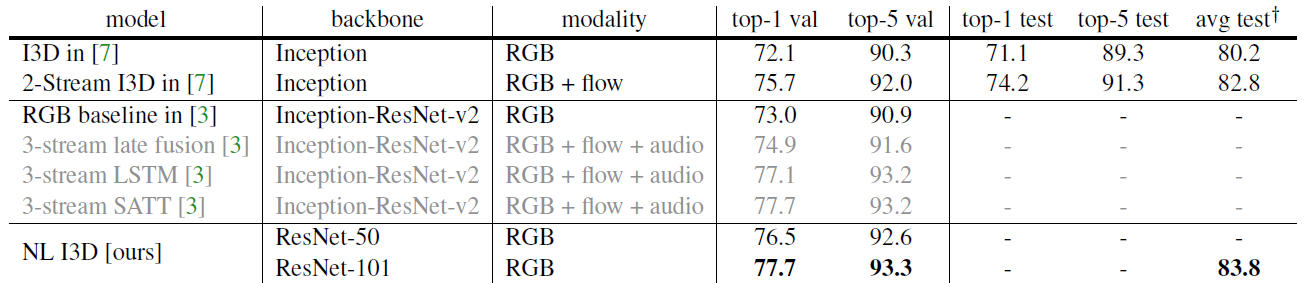Comparisons with state-of-the-art results in Kinetics, reported on the val and test sets
• The proposed method surpasses all the existing RGB or RGB + flow based methods by a good margin.
• Without using optical flow and without any bells and whistles, the proposed method is on par with the heavily engineered results of the 2017 competition winner.

• Charades  is a multi-label video dataset with 8k training, 1.8k validation, and 2k testing videos, with 157 action categories.
• A per-category sigmoid output is used here.
• The proposed I3D baseline is higher than the previous results.
• As a controlled comparison, the proposed non-local net improves over our I3D baseline by 2.3% on the test set.

# 7. Experimental Results on COCO

• The Mask R-CNN baseline is used for COCO object detection, segmentation and human pose estimation (keypoint detection).
• All backbones are used with FPN.
• The models are trained on COCO train2017 (i.e., trainval35k in 2014) and tested on val2017 (i.e., minival in 2014).

## 7.1. Object Detection and Instance Segmentation

• R50/R101 is ResNet-50/101, and X152 is ResNeXt-152.
• A single non-local block improves all R50/101 and X152 baselines.
• This comparison suggests that non-local dependency has not been sufficiently captured by existing models despite increased depth/capacity.
• The above gain is at a very small cost. The single non-local block only adds <5% computation to the baseline model.
• Authors also have tried to use more non-local blocks to the backbone, but found diminishing return.

## 7.2. Keypoint Detection

• Mask R-CNN used a stack of 8 convolutional layers for predicting the keypoints as 1-hot masks. These layers are local operations and may overlook the dependency among keypoints across long distance.
• 4 non-local blocks are inserted into the keypoint head (after every 2 convolutional layers).
• On a strong baseline of R101, adding 4 non-local blocks to the keypoint head leads to a 1 point increase of keypoint AP.
• If one extra non-local block is added to the backbone as done for object detection, in total 1.4 points increase of keypoint AP over the baseline is observed.

## Reference

[2018 CVPR] [NL: Non-Local Neural Networks]
Non-local Neural Networks

## Video Classification

2014 [Deep Video] [Two-Stream ConvNet] 2015 [DevNet] [C3D] 2016 [TSN] 2017 [Temporal Modeling Approaches] [4 Temporal Modeling Approaches] [P3D] 2018 [NL: Non-Local Neural Networks]

## More from Sik-Ho Tsang

PhD, Researcher. I share what I've learnt and done. :) My LinkedIn: https://www.linkedin.com/in/sh-tsang/, My Paper Reading List: https://bit.ly/33TDhxG

Love podcasts or audiobooks? Learn on the go with our new app.

## Create Machine Learning Model Inside Docker## PyTorch Multi-GPU Metrics and more in PyTorch Lightning 0.8.1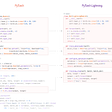## Build a Multi-Layer Perceptron (Backpropagation) for a Bank Marketing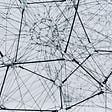## Review — ALI: Adversarially Learned Inference (GAN)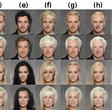## Review — SepConv++: Revisiting Adaptive Convolutions for Video Frame Interpolation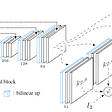## Short Introduction To PyTorch## Parts of Speech Tagging with Hidden Markov Model## Sik-Ho Tsang

PhD, Researcher. I share what I've learnt and done. :) My LinkedIn: https://www.linkedin.com/in/sh-tsang/, My Paper Reading List: https://bit.ly/33TDhxG

## Review — Image Style Transfer Using Convolutional Neural Networks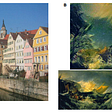## Image Classification with ResNets in PyTorch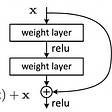## Neural Style Transfer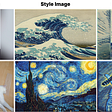## AnimeGAN: a GAN for style transfer